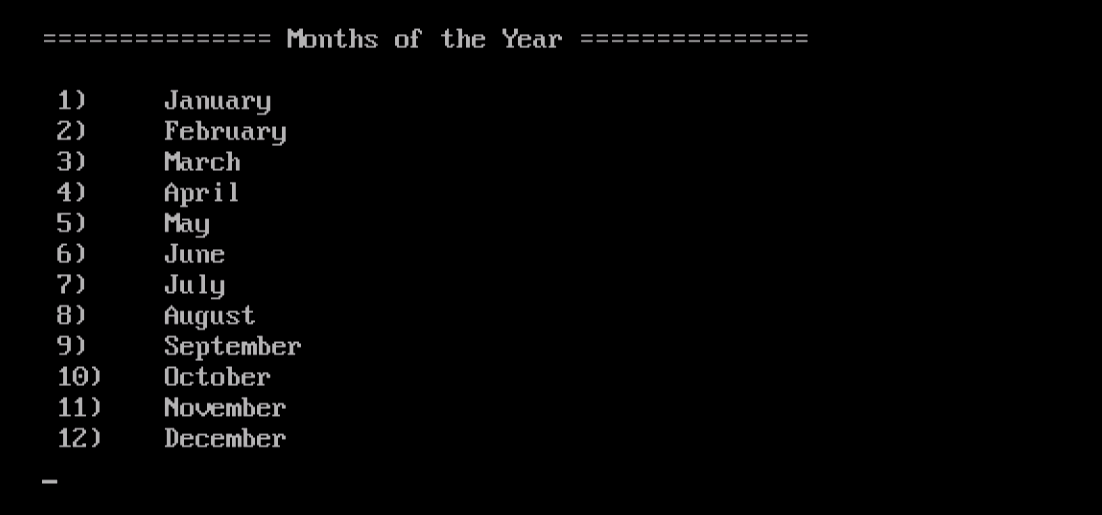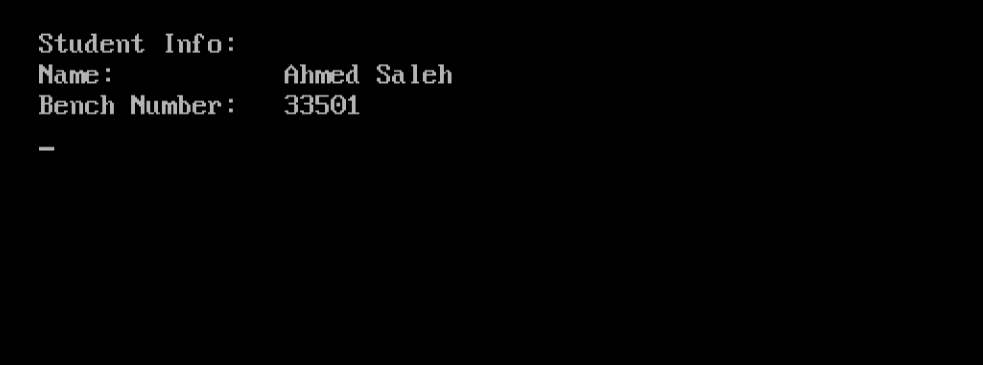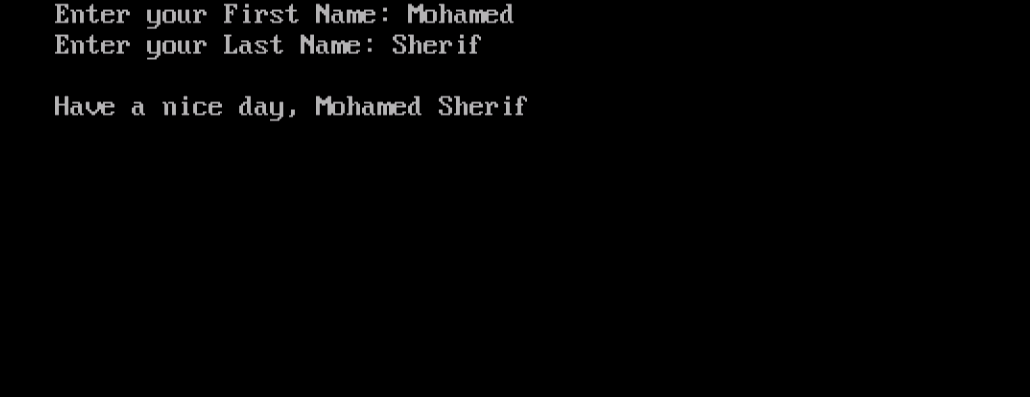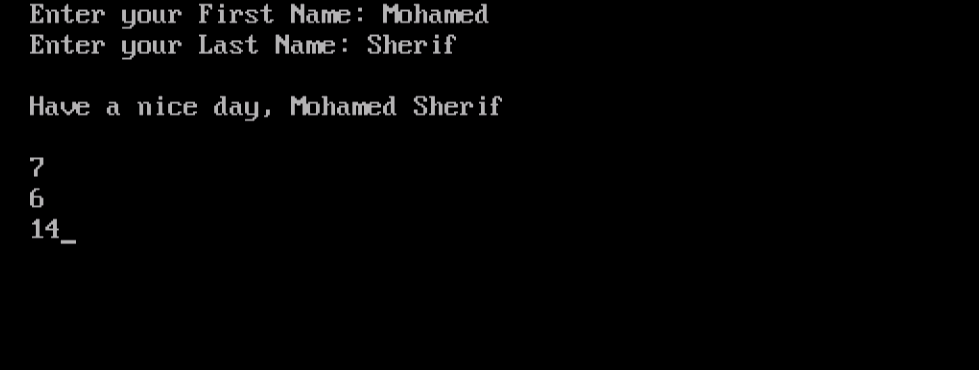System ProgrammingLearn strcpy, strcat and strlen function in C++ Programming

# Learn strcpy, strcat and strlen function in C++ Programming

In the previous article, we had an introduction to Strings. We have learned the original implementation of strings as arrays of characters. In this article, we are going to utilize what we have learned in the previous one, and build upon.

Using Arrays of Strings
C++ supports multi-dimensional arrays. The simplest form of this type is the two-dimensional array. A two dimensional-array is an array of arrays; i.e. each element of the array is itself an array. Following this definition, and given that a string is an array of characters, we can conclude that an array of strings is actually a two-dimensional array of characters.

To define an array of strings, use the following syntax:

`char STRARRAY[ROWS][MAXSTRLENGTH];`

Where:
STRARRAY is the name of the array of strings. It could be any valid C++ identifier.
ROWS is the number of elements (strings) that the array contains.
MAXSTRLENGTH is the maximum possible length of the strings.

Example
The following program stores the month names in an array of strings called months.

```#include <iostream.h>
#include <conio.h>
void main()
{
clrscr();
char months = { "January", "February", "March", "April",
"May", "June", "July", "August", "September",
"October", "November", "December" };
cout << "\n=============== Months of the Year ===============\n\n";
for(int i=0;i<12;i++)
cout << " " << i+1 << ")\t" << months[i] << endl;
getch();
}
```

When executed, we should get the following output:In the array definition:

`char months = { … };`
• The number 12 is the number of elements (strings) in the outer array. This is self-explanatory: 12 elements for 12 months.
• The number 10 is the maximum length an individual string could be. Although the longest month name is 9 letters (September), the terminating null character ‘\0’ makes the required number of (inner) character array elements 10.

Useful String Functions
C++ has a rich set of functions that manipulate strings. In this section, we are going to investigate some of these functions and their uses, and will illustrate our talk with examples.

The strcpy() Function
This function copies a string from source to destination. The function takes two string variables as arguments: the destination, and the source, then returns the updated destination variable.

Example
The following program defines a class named student, which has two member data items: name and benchnum. The constructor uses the strcpy() function to initialize the name variable in new objects.

```#include <iostream.h>
#include <conio.h>
#include <string.h>
class student
{
char name;
long benchnum;
public:
student(char sname[],long bnum)
{
strcpy(name,sname);  	//Remember: strcpy (dst, src);
benchnum = bnum;
}
char * getname()
{ return name; }
long getbenchnum()
{ return benchnum; }
};
void main()
{
clrscr();
student s1("Ahmed Saleh", 33501);
cout << "\nStudent Info:\n"
<< "Name:\t\t"   << s1.getname() << endl
<< "Bench Number:\t" << s1.getbenchnum() << endl;
getch();
}
```

That is what we get when executing this code:There are two points that need some explanation in this program:

• First: the use of the string.h header file, which is necessary for the program to compile successfully, and not complain about the strcpy() function.
• Second: in the member function definition:
`char * getname()`

The expression char *  (in the return value type) is another method to define strings. We will talk about this method in more details later in this series when talking about Pointers. But for now, remember that char * defines a string.The strcat() Function
The strcat() function concatenates two strings. It appends a copy of the source string to the end of the destination string, and then returns the destination string.

This function also require including the string.h library file.

Example
The following example concatenates the user’s first and last names into a string variable fullname, and prints it.

```#include <iostream.h>
#include <conio.h>
#include <string.h>
void main()
{
clrscr();
char first,last;
char * fullname;
cout << "Enter your First Name: "; cin >> first;
cout << "Enter your Last Name: "; cin >> last;
strcpy(fullname,first);
strcat(fullname," ");
strcat(fullname,last);
cout << "\nHave a nice day, " << fullname;
getch();
}
```

This program should behave like this:After receiving the user’s input (first and last), the program copies the string variable first (first name) into the variable fullname:

```strcpy(fullname,first);

```

Now, the fullname contains the first name. Next, the program uses the strcat() function to append a white space after the first name into the fullname string variable:

`strcat(fullname," ");`

Finally, the last name is appended to fullname string variable:

`strcat(fullname,last);`

The strlen() Function
I know you have been waiting for this!!! This function calculates the length of  string.

Example
In the previous string concatenation example, consider adding the following line of code just before the getch() function at the end of the main() function:

```cout 	<< endl << strlen(first) << endl << strlen(last)
<< endl << strlen(fullname);
```

This line will print the lengths of the string variables first, last, and fullname, respectively.Summary
In this article, we have continued our talk about Strings.

• You can define an array of strings. In its reality, it is a two-dimensional array (array of arrays).
• C++ has a rich set of useful functions that manipulate strings, like strcpy(), strcat(), and strlen().

In the next article, we are going to learn more string functions. See you there.

### Build A Front End Of an e-Commerce Site Using React

21,501Fans
4,210Followers
104,000Subscribers

### Build A Front End Of an e-Commerce Site Using React

- Advertisement -